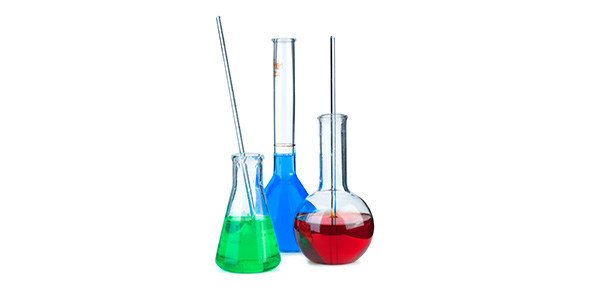# Remedial Kesetimbangan Kimia

5 Questions | Total Attempts: 39SettingsREMEDIAL UH 5

Related Topics
• 1.
• The reaction of methane:
C(s)  +  2H2(g)                        CH4(g)   ΔH = -18kj The methane produced will increase if:
• The temperature is decreased
• The temperature is increased
• The pressure is decreased
• The pressure is increased
The correct statement are…
• A.

1, 2 and 3

• B.

1 and 4

• C.

1 and 2

• D.

2 and 4

• E.

All the correct

• 2.
• Five mole ammonia gas is put into a container 3L and left dissociate based on the reaction:
2NH3(g)                         N2(g) +  3H2(g) After the equilibrium is reached, 40% of the ammonia is dissociated and causes the total pressure as 3,5 atm. The value of  Kp of the reaction is….
• A.

0.5

• B.

0.75

• C.

1

• D.

1.5

• E.

1.75

• 3.
• In a closed container, 3M of gas A react with 3M gas B and forms 2M gas C based on the reaction:
A(g) + 2B(g)               2C(g). If the total pressure of the system is 2 atm, the value of the Kp is….
• A.

5

• B.

0.5

• C.

0.75

• D.

0.05

• E.

0.25

• 4.
COCl2(g)              CO(g) + Cl2(g) And in equilibrium there is 0,1M COCl2. The value of Kc is…..
• A.

1

• B.

1.6

• C.

2.6

• D.

2

• E.

3

• 5.
• At the equilibrium reaction
N2(g) + 3H2(g)             2NH3(g)  ΔH = -92kj The equilibrium will shift to the product if….
• A.

Temperature is decreased

• B.